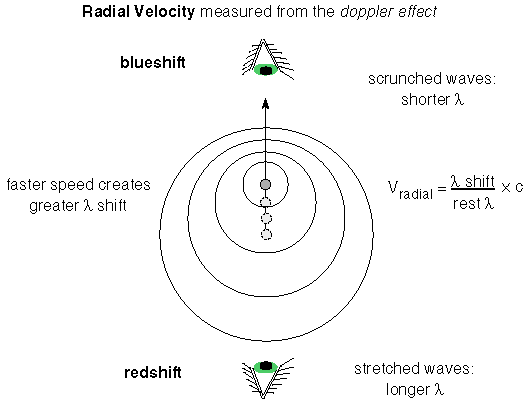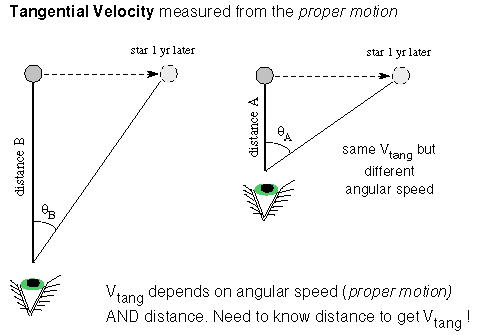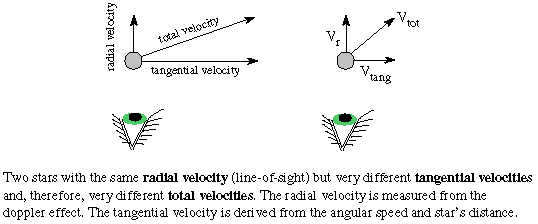### The Velocities of Stars

#### Chapter index in this window —   — Chapter index in separate window

This material (including images) is copyrighted!. See my copyright notice for fair use practices.

The velocity of stars are determined by using the doppler effect (see the doppler effect section). The motion of a star causes a shift in the wavelengths received. The star emits the same spectrum it would emit if it was at rest. The pattern of lines depends on the internal physics of the star itself. The doppler effect depends on the external property of the star's motion through space relative to us. The wavelength shift=new -rest, wherenew is the observed wavelength andrest is the wavelength you would observe if the star was not moving relative to us. The amount of the shift depends on the velocity of the star in this way:/rest = (radial velocity) / c, where c is the speed of light and the radial velocity is the component of the star's motion that is along the line of sight. Rearranging the terms you find the radial velocity = (/rest) × c.If the star is moving away from you, then you see the wavelengths stretched out andis a positive number. The spectral lines appear shifted to the red end of the spectrum, so the shift is called a redshift. If the star is moving toward you, then the wavelengths appear to the compressed andis a negative number. The spectral lines are shifted to the blue end of the spectrum, so the shift is called a blueshift. The doppler effect tells you only about the motion relative to you. If you move toward the star or it moves toward you, the doppler effect will be the same. The Earth is in motion relative to the star because it are orbiting the Sun, so you have to take the Earth's orbital motion into account when figuring the star's velocity from the doppler effect.

Also, the doppler effect gives you only the speed along the line of sight. Most stars move at an angle to our line of sight. The part of a star's total velocity that is perpendicular to the line of sight is called the tangential velocity.To get the tangential velocity, you need to first measure the angular velocity of the star across the sky (d/dt). This is how many degrees on the sky the star moves in a given amount of time and is called the proper motion by astronomers. If you determine the star's distance from its trigonometric parallax or the inverse square law method, you can convert the angular velocity (proper motion) to tangential velocity in physical units such as kilometers/second. The tangential velocity = k × the star's distance × the proper motion, where k is a conversion factor that will take care of the conversion from arc seconds and parsecs and years to kilometers/second. Using the Pythagorean theorem for right triangles, you find that the star's total velocity = Sqrt[(radial velocity)2 + (tangential velocity)2].#### Go to Astronomy Notes home

last updated: June 2, 2007